2020-03-09 16:44:42 qq_36573282 阅读数 37
• ###### C++语音识别开篇

本篇mark老师将教大家使用第三方库的调用来简单的实现语音识别。随着机器学习和人工智能的热闹，国内语音行业也可谓是百花齐放。 语音识别一个伟大的时代已在我们身边悄悄走来。

6112 人正在学习 去看看 杨波

# 数字语音识别的基本步骤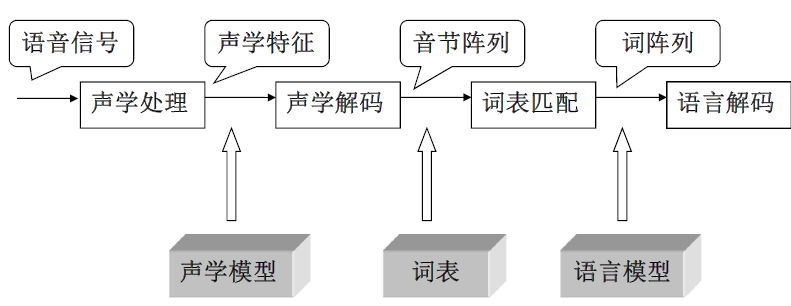# 语音识别模型

## 隐马尔可夫模型

1. 评估：给定观测向量Y和模型，利用前向后向算法计算得分；
2. 匹配：给定观测向量Y，用Viterbi算法确定一个优化的状态序列；
3. 训练：用Baum-Welch算法(类似于EM)重新估计参数，使得得分最大。
已知一个有限的离散状态序列$S=\{q_1,q_2,\dots,q_{N-1},q_N\}$，从时间$t$到时间$t+1$，保持当前状态或迁移到另一个状态。从时刻$t$状态为$q_i$迁移到时刻$t+1$状态为$q_j$，概率为$a_{ij}=P(q_{t+1}=j|q_t=i),1\leq i,j\leq N$。这样就可以得到状态之间的迁移概率矩阵。
$A=\left[\begin{matrix} a_{11} & a_{12} & \dots & a_{1N}\\ a_{21} & a_{22} & \dots & a_{2N}\\ \dots & \dots & \dots & \dots\\ a_{N1} & a_{N2} & \dots & a_{NN} \end{matrix}\right]$
在矩阵$A$中，$a_{ij}$满足$a_{ij}\geq0, \forall ij$，且$\sum_{j=1}^{N}a_{ij}=1, \forall i$
马尔可夫有两个基本假设：一是过程是无记忆的，即$P(q_{t+1}=j|q_t=i,q_{t-1}=k,\dots)=P(q_{t+1}=j|q_{t}=i)$；二是马尔可夫过程是时间同质(homogeneous)的，即$P(q_{t+1}=j|q_t=i)=P(q_{t+1+k}=j|q_{t+k}=i)$
在计算某个序列的概率的时候，可以利用马尔可夫的链式规则来计算。例如，计算序列$q_1,q_2,\cdots, q_T$的概率，
\begin{aligned}&P(q_1, q_2,\dots, q_T)\\ &=P(q_T|q_1,q_2,\dots,q_{T-1})P(q_1,q_2,\dots,q_{T-1})\\ &=P(q_T|q_{T-1})P(q_1,q_2,\dots,q_{T-1})\\ &=\cdots\\ &=P(q_T|q_{T-1})P(q_{T-1}|q_{T-2})\cdots P(q_2|q_1)P(q_1) \end{aligned}
上面都是离散状态的隐马尔可夫模型，对于连续的马尔可夫模型，观察值是连续的随机变量$X$。某个状态$j$对应的观察值统计特性由一个观察值概率密度函数$b_{j}(X)$表示。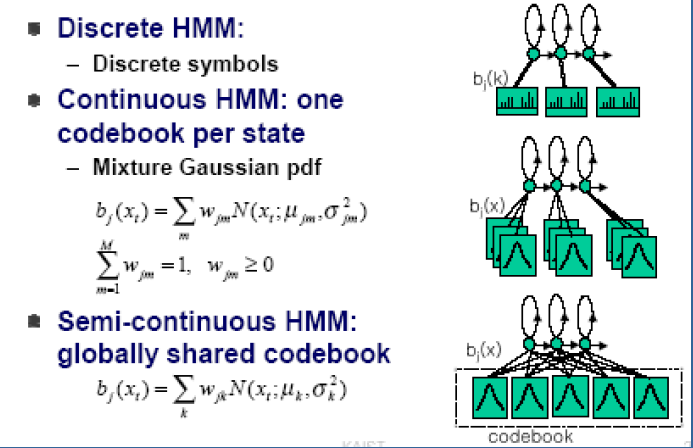## 前向后向算法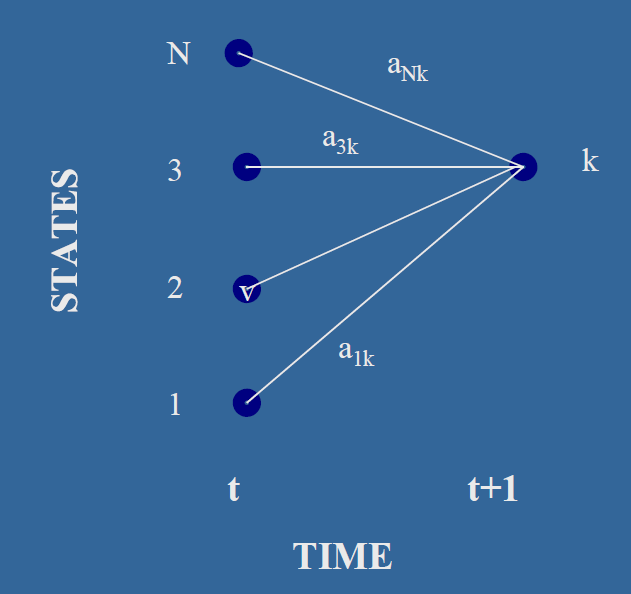## 维特比算法

1. 初始化：$\delta_1(i)=\pi_ib_i(O_1), 1 \leq i \leq N$, $\psi_1(i)=0$
2. 递推过程：$\delta_t(j)=max_{1\leq i \leq N}[\delta_{t-1}(i)\alpha_{ij}]b_i(O_t),2 \leq t \leq T, 1 \leq j \leq N$,
$\psi_t(j)=argmax_{1\leq i \leq N}[\delta_{t-1}(i)\alpha_{ij}], 2\leq t \leq T, 1 \leq j \leq N$
3. 终止：$P^*=max_{1\leq i \leq N}[\delta_T(i)]$,
$q^*_T=argmax_{1\leq i \leq N}[\delta_T(i)]$

• 初始化模型参数 $\lambda_0$;
• 使用观测序列$O$和模型参数$\lambda$得到新的模型$\widetilde{\lambda}$;
• $\widetilde{\lambda} \leftarrow \lambda$;
• 重复步骤2-3，直到 $logP(O|\lambda)-logP(O|\lambda_0)\lt d$

2019-08-01 11:32:20 weixin_40796925 阅读数 1584
• ###### C++语音识别开篇

本篇mark老师将教大家使用第三方库的调用来简单的实现语音识别。随着机器学习和人工智能的热闹，国内语音行业也可谓是百花齐放。 语音识别一个伟大的时代已在我们身边悄悄走来。

6112 人正在学习 去看看 杨波

# 语音识别API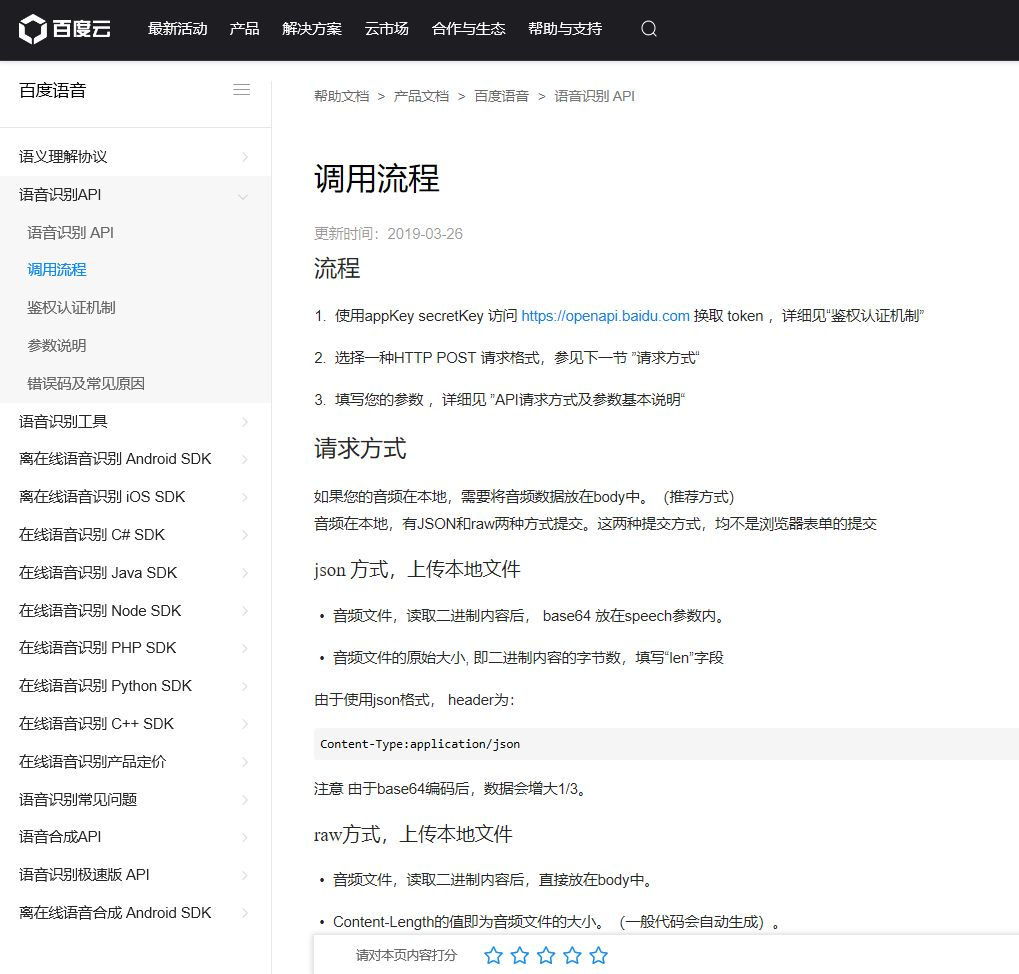# 语音识别步骤

1. 先注册百度云的账号，控制台中创建百度语音的应用，获取API Key和Secret Key
2. 通过API Key 和 Secret Key获取token
3. 将token和本地音频数据上传到API链接
4. 根据API返回结果获取解析后的文字结果

# 效果展示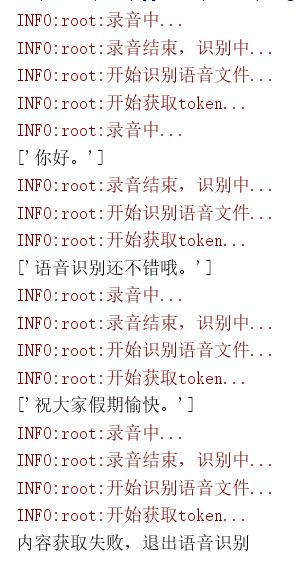# 代码下载

GitHub代码下载：
https://github.com/pengfexue2/yuyin.git2018-08-08 15:26:48 qq_42749116 阅读数 3478
• ###### C++语音识别开篇

本篇mark老师将教大家使用第三方库的调用来简单的实现语音识别。随着机器学习和人工智能的热闹，国内语音行业也可谓是百花齐放。 语音识别一个伟大的时代已在我们身边悄悄走来。

6112 人正在学习 去看看 杨波

我们的语音文件在很多的时候都会进行识别，因为语音在某些场合不适合听，我们就会转换成语音尽心，但是你在使用的语音识别软件可以识别完全的语音吗？识别出来的文字会和语音的内容相同吗？其实很多的语音识别软件都会都这种错误，识别不出来语音的面的某些字，今天给大家推荐一款在线进行语音识别的软件——迅捷PDF在线转换器，我们一起来操作一下吧。

步骤一：打开浏览器，搜索迅捷PDF在线转换器，点击进入网站首页。步骤二：进入网站首页，在导航栏的位置找到语音识别，把鼠标移动到语音识别，会看到语音转文字。步骤三：进入转换页面，点击选择文件，选择需要进行识别的语音文件。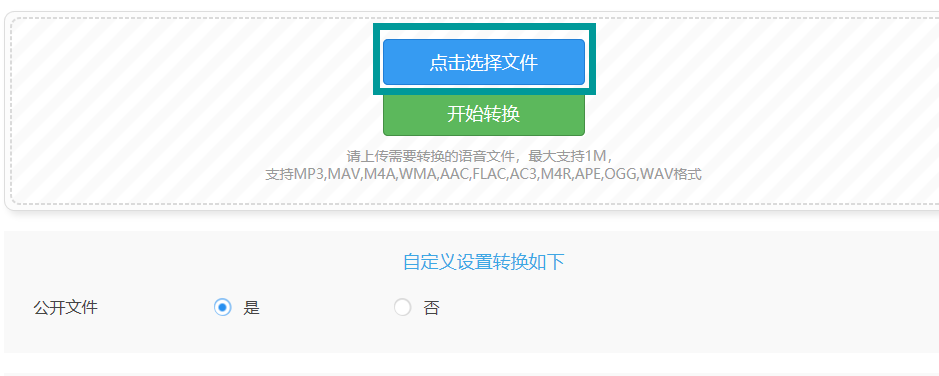步骤四：添加完文件以后，点击开始转换，需要识别的语音文件就会在识别的过程中，请耐心等待。步骤五：转换完成的文件可以直接进行下载，也会在导航栏上方的我的文件里进行保存。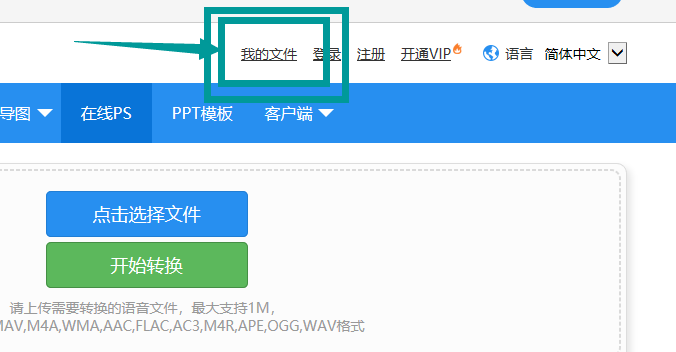以上就是进行语音识别的软件，以及语音识别的步骤。

2016-11-24 16:25:52 KwunYamShan 阅读数 6169
• ###### C++语音识别开篇

本篇mark老师将教大家使用第三方库的调用来简单的实现语音识别。随着机器学习和人工智能的热闹，国内语音行业也可谓是百花齐放。 语音识别一个伟大的时代已在我们身边悄悄走来。

6112 人正在学习 去看看 杨波

#科大讯飞-语音识别开发

##在线语音识别

语音识别在模拟器上是无法测试的，虚拟机mac接收不到声音或者语音识别是访问云端数据，所以如果手机的网络没有开启，就无法实现识别声音的！一定要开启手机的网络，如果手机不存在语音识别功能的话，也是无法启用识别！


##效果图##源码

##集成步骤

Step 1. 导入so库

eclipse将so库导入到libs目录下
AS用户将so导入到jniLibs目录下
下载的官方SDK中有七个so库目前已经支持市面上98%的手机
armeabi目录下libmsc.so只支持市面上百分之八十的手机，如果不需要做适配的话导入这一个so库也是极好的Step 2. 拷贝资产文件
将 SDK 资源包assets 路径下的资源文件拷贝至 Android 工程 asstes 目录下Step 3.  初始化
这是很重要并且是必须的一步操作,实际上初始化的操作是异步进行的,如果初始化还没有完成你就开始调用了语音识别,是会出现很多问题的,建议在你程序刚刚启动的时候调用

public class SpeechApp extends Application {

@Override
public void onCreate() {
// 应用程序入口处调用，避免手机内存过小，杀死后台进程后通过历史intent进入Activity造成SpeechUtility对象为null
// 如在Application中调用初始化，需要在Mainifest中注册该Applicaiton
// 参数间使用半角“,”分隔。
// 设置你申请的应用appid,请勿在'='与appid之间添加空格及空转义符

// 注意： appid 必须和下载的SDK保持一致，否则会出现10407错误

SpeechUtility.createUtility(SpeechApp.this, "appid=" + getString(R.string.app_id));

// 以下语句用于设置日志开关（默认开启），设置成false时关闭语音云SDK日志打印
// Setting.setShowLog(false);
super.onCreate();
}

}

Step 4.  添加用户权限，官方给的API中不规范有几条权限中间有空格导致编译不通过 ,注意一下

<!--连接网络权限，用于执行云端语音能力 -->
<uses-permission android:name="android.permission.INTERNET"/>
<!--获取手机录音机使用权限，听写、识别、语义理解需要用到此权限 -->
<uses-permission android:name="android.permission.RECORD_AUDIO"/>
<!--读取网络信息状态 -->
<uses-permission android:name="android.permission.ACCESS_NETWORK_STATE"/>
<!--获取当前wifi状态 -->
<uses-permission android:name="android.permission.ACCESS_WIFI_STATE"/>
<!--允许程序改变网络连接状态 -->
<uses-permission android:name="android.permission.CHANGE_NETWORK_STATE"/>
<!--读取手机信息权限 -->
<!--读取联系人权限，上传联系人需要用到此权限 -->
<!--外存储写权限， 构建语法需要用到此权限 -->
<uses-permission android:name="android.permission.WRITE_EXTERNAL_STORAGE"/>
<!--外存储读权限，构建语法需要用到此权限 -->
<!--配置权限，用来记录应用配置信息 -->
<uses-permission android:name="android.permission.WRITE_SETTINGS"/>
<!--手机定位信息，用来为语义等功能提供定位， 提供更精准的服务-->
<!--定位信息是敏感信息， 可通过Setting.setLocationEnable(false)关闭定位请求 -->
<uses-permission android:name="android.permission.ACCESS_FINE_LOCATION"/>
<!--如需使用人脸识别，还要添加：摄相头权限， 拍照需要用到-->
<uses-permission android:name="android.permission.CAMERA" />

Step 5. 代码

public class MainActivity extends Activity {

private StringBuffer mBuffer;
private TextView tv;

@Override
protected void onCreate(Bundle savedInstanceState) {
super.onCreate(savedInstanceState);
setContentView(R.layout.activity_main);
tv = (TextView) findViewById(R.id.tv_translate_text);
}

public void startVoice(View view) {
//1.创建RecognizerDialog对象
RecognizerDialog mDialog = new RecognizerDialog(this, null);
//2.设置accent、 language等参数
mDialog.setParameter(SpeechConstant.LANGUAGE, "zh_cn");
mDialog.setParameter(SpeechConstant.ACCENT, "mandarin");
//若要将UI控件用于语义理解，必须添加以下参数设置，设置之后onResult回调返回将是语义理解结果
// mDialog.setParameter("asr_sch", "1");
// mDialog.setParameter("nlp_version", "2.0");

mBuffer = new StringBuffer();
//3.设置回调接口
mDialog.setListener(mRecognizerDialogListener);
//4.显示dialog，接收语音输入
mDialog.show();
}

RecognizerDialogListener mRecognizerDialogListener = new RecognizerDialogListener() {
//听写结果回调接口(返回Json格式结果，用户可参见附录13.1)；
//一般情况下会通过onResults接口多次返回结果，完整的识别内容是多次结果的累加；
//关于解析Json的代码可参见Demo中JsonParser类；
//isLast等于true时会话结束。
@Override
public void onResult(RecognizerResult results, boolean isLast) {
String result = results.getResultString();//语音听写的结果

String resultString = processData(result);

mBuffer.append(resultString);

if (isLast) {
//话已经说完了
String finalResult = mBuffer.toString();
System.out.println("解析结果:" + finalResult);
tv.setText(finalResult);

}

}

@Override
public void onError(SpeechError error) {

}
};

//解析json
protected String processData(String result) {
Gson gson = new Gson();
VoiceBean voiceBean = gson.fromJson(result, VoiceBean.class);

StringBuffer sb = new StringBuffer();

ArrayList<VoiceBean.WsBean> ws = voiceBean.ws;
for (VoiceBean.WsBean wsBean : ws) {
String word = wsBean.cw.get(0).w;
sb.append(word);
}

return sb.toString();
}

}



layout

<?xml version="1.0" encoding="utf-8"?>
<LinearLayout xmlns:android="http://schemas.android.com/apk/res/android"
android:orientation="vertical" android:layout_width="match_parent"
android:layout_height="match_parent"

>
<TextView
android:layout_width="match_parent"
android:layout_height="230dip"
android:id="@+id/tv_translate_text"
android:background="#fff"
android:textSize="22sp"
android:textColor="#000"
android:hint="请点击按钮"
/>
<LinearLayout
android:layout_width="match_parent"
android:layout_height="match_parent"
android:orientation="horizontal"
android:background="#F1AF5A"
android:gravity="center"
>
<ImageButton
android:layout_width="wrap_content"
android:layout_height="wrap_content"
android:background="@drawable/button"
android:onClick="startVoice"
/>
</LinearLayout>

</LinearLayout>

Step 6. 目录结构